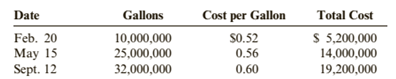Chapter 6, Problem 70APSA### Cornerstones of Financial Accounti...

4th Edition
Jay Rich + 1 other
ISBN: 9781337690881

#### Solutions

Chapter
Section### Cornerstones of Financial Accounti...

4th Edition
Jay Rich + 1 other
ISBN: 9781337690881
Textbook Problem
1 views

# Inventory Costing and LCMOrtman Enterprises sells a chemical used in various manufacturing processes. On January 1, 2019, Ortman had 5,000,000 gallons on hand, for which it had paid $0.50 per gallon. During 2019, Ortman made the following purchases:During 2019, Ortman sold 65 000,000 gallons at$0.75 per gallon (35,000,000 gallons were sold on June 29 and 30,000,000 gallons were sold on Nov. 22), leaving an ending inventory of 7,000,000 gallons. Assume that Ortman uses a perpetual inventory system. Ortman uses the lower of cost or market for its inventories, as required by generally accepted accounting principles.Required:1. Assume that the market value of the chemical is $0.76 per gallon on December 31, 2019. Compute the cost of ending inventory using the FIFO and average cost methods, and then apply LCM. ( Note: Use four decimal places for per-unit calculations and round all other numbers to the nearest dollar.)2. Assume that the market value of the chemical is$0.58 per gallon on December 31, 2019. Compute the cost of ending inventory using the FIFO and average cost methods, and then apply LCM. ( Note: Use four decimal places for per-unit calculations and round all other numbers to the nearest dollar.)

To determine

(a)

Lower of cost or market value:

Usually the businesses follow the historical cost principle in which the inventory is valued at the cost of the purchase. But in some instances, it has been seen that the market value falls below the cost due to its obsolescence or damaged nature. In those cases, the businesses follow the principle to value the inventory at lower of cost or market value.

The cost of ending inventory using the FIFO and other cost methods and then apply LCM with given market value of 0.76 per gallon.

Explanation

The given information for the year 2019 is as follows:

Total available gallons are:

Opening inventory=5000000 gallons @ $0.50 each Purchases=10000000 gallons @$0.52 eachPurchases=25000000 gallons @ $0.56 eachPurchases=32000000 gallons @$0.60 each

Total Purchased gallons = 67000000

Total available gallons = 5000000+67000000=72000000 gallons

Sales=35000000+30000000 gallons=65000000 gallons

Closing inventory=7000000 gallons

The given market value in the question is of 0.76 per gallon.

Calculation of Closing Inventory as per FIFO Method:

Under this method, which material purchased first, issued first for production. However closing inventory includes last purchased materials in stock. Due to latest purchase in closing inventory, higher value of latest purchase effects cost of goods sold as lower and profit margin will be high.

 Closing inventory Cost of Goods sold 5000000 @$0.50 =$2500000 10000000 @$0.52 =$5200000 20000000 @$0.56=$11200000 5000000 @$0.56=$2800000 7000000 @$0.60=$4200000 25000000 @$0.60=$15000000 Total 7000000 @$0.60=$4200000

As the cost of closing inventory is $0.60 per gallon which is lower than the market value given in the question i.e. 0.76 per gallon. Hence, the valuation of closing inventory is to be done on the cost only. Calculation of closing inventory as per LIFO Method: Under this method, which material purchased last, issued first for production. However closing inventory includes earliest purchased material in stock. Due to earliest purchase material in stock, lower value of earliest purchased effects cost of goods sold as high and profit margin will be lower.  Closing inventory Cost of Goods sold 25000000 @$0.56 = $14000000 10000000 @$0
To determine

(b)

Lower of cost or market value:

Usually the businesses follow the historical cost principle in which the inventory is valued at the cost of the purchase. But in some instances, it has been seen that the market value falls below the cost due to its obsolescence or damaged nature. In those cases, the businesses follow the principle to value the inventory at lower of cost or market value.

The cost of ending inventory using the FIFO and other cost methods and then apply LCM with given market value of 0.58 per gallon.

### Still sussing out bartleby?

Check out a sample textbook solution.

See a sample solution

#### The Solution to Your Study Problems

Bartleby provides explanations to thousands of textbook problems written by our experts, many with advanced degrees!

Get Started

#### What is sparse data? Give an example.

Database Systems: Design, Implementation, & Management

#### Solve Problem 20.21 using Excel.

Engineering Fundamentals: An Introduction to Engineering (MindTap Course List)

#### What is a project champion?

Management Of Information Security